### SOLUTIONS TO IMPLICIT DIFFERENTIATION PROBLEMS

SOLUTION 1 : Begin with x3 + y3 = 4 . Differentiate both sides of the equation, getting

D ( x3 + y3 ) = D ( 4 ) ,

D ( x3 ) + D ( y3 ) = D ( 4 ) ,

(Remember to use the chain rule on D ( y3 ) .)

3x2 + 3y2 y' = 0 ,

so that (Now solve for y' .)

3y2 y' = - 3x2 ,

and.

SOLUTION 2 : Begin with (x-y)2 = x + y - 1 . Differentiate both sides of the equation, getting

D (x-y)2 = D ( x + y - 1 ) ,

D (x-y)2 = D ( x ) + D ( y ) - D ( 1 ) ,

(Remember to use the chain rule on D (x-y)2 .),

2 (x-y) (1- y') = 1 + y' ,

so that (Now solve for y' .)

2 (x-y) - 2 (x-y) y' = 1 + y' ,

- 2 (x-y) y' - y' = 1 - 2 (x-y) ,

(Factor out y' .)

y' [ - 2 (x-y) - 1 ] = 1 - 2 (x-y) ,

and.

SOLUTION 3 : Begin with. Differentiate both sides of the equation, getting,

(Remember to use the chain rule on.),,

so that (Now solve for y' .),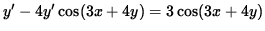,

(Factor out y' .),

and.

SOLUTION 4 : Begin with y = x2 y3 + x3 y2 . Differentiate both sides of the equation, getting

D(y) = D ( x2 y3 + x3 y2 ) ,

D(y) = D ( x2 y3 ) + D ( x3 y2 ) ,

(Use the product rule twice.),

(Remember to use the chain rule on D ( y3 ) and D ( y2 ) .)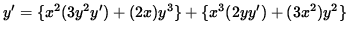,

y' = 3x2 y2 y' + 2x y3 + 2x3 y y' + 3x2 y2 ,

so that (Now solve for y' .)

y' - 3x2 y2 y' - 2x3 y y' = 2x y3 + 3x2 y2 ,

(Factor out y' .)

y' [ 1 - 3x2 y2 - 2x3 y ] = 2x y3 + 3x2 y2 ,

and.

SOLUTION 5 : Begin with. Differentiate both sides of the equation, getting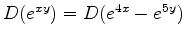,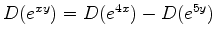,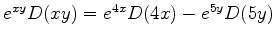,,

so that (Now solve for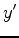.)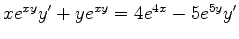,,

(Factor out.)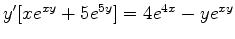,

and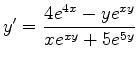.

SOLUTION 6 : Begin with. Differentiate both sides of the equation, getting,,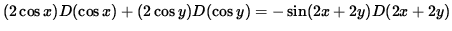,,

so that (Now solve for y' .),,

(Factor out y' .),,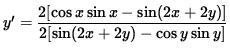,

and.

SOLUTION 7 : Begin with. Differentiate both sides of the equation, getting,

1 = (1/2)( x2 + y2 )-1/2 D ( x2 + y2 ) ,

1 = (1/2)( x2 + y2 )-1/2 ( 2x + 2y y' ) ,

so that (Now solve for y' .),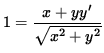,,,

and.

SOLUTION 8 : Begin with. Clear the fraction by multiplying both sides of the equation by y + x2 , getting,

or

x - y3 = xy + 2y + x3 + 2x2 .

Now differentiate both sides of the equation, getting

D ( x - y3 ) = D ( xy + 2y + x3 + 2x2 ) ,

D ( x ) - D (y3 ) = D ( xy ) + D ( 2y ) + D ( x3 ) + D ( 2x2 ) ,

(Remember to use the chain rule on D (y3 ) .)

1 - 3 y2 y' = ( xy' + (1)y ) + 2 y' + 3x2 + 4x ,

so that (Now solve for y' .)

1 - y - 3x2 - 4x = 3 y2 y' + xy' + 2 y' ,

(Factor out y' .)

1 - y - 3x2 - 4x = (3y2 + x + 2) y' ,

and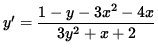.

SOLUTION 9 : Begin with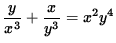. Clear the fractions by multiplying both sides of the equation by x3 y3 , getting,,

y4 + x4 = x5 y7 .

Now differentiate both sides of the equation, getting

D ( y4 + x4 ) = D ( x5 y7 ) ,

D ( y4 ) + D ( x4 ) = x5 D (y7 ) + D ( x5 ) y7 ,

(Remember to use the chain rule on D (y4 ) and D (y7 ) .)

4 y3 y' + 4 x3 = x5 (7 y6 y' ) + ( 5 x4 ) y7 ,

so that (Now solve for y' .)

4 y3 y' - 7 x5 y6 y' = 5 x4 y7 - 4 x3 ,

(Factor out y' .)

y' [ 4 y3 - 7 x5 y6 ] = 5 x4 y7 - 4 x3 ,

and.

SOLUTION 10 : Begin with (x2+y2)3 = 8x2y2 . Now differentiate both sides of the equation, getting

D (x2+y2)3 = D ( 8x2y2 ) ,

3 (x2+y2)2 D (x2+y2) = 8x2 D (y2 ) + D ( 8x2 ) y2 ,

(Remember to use the chain rule on D (y2 ) .)

3 (x2+y2)2 ( 2x + 2 y y' ) = 8x2 (2 y y' ) + ( 16 x ) y2 ,

so that (Now solve for y' .)

6x (x2+y2)2 + 6 y (x2+y2)2 y' = 16 x2 y y' + 16 x y2 ,

6 y (x2+y2)2 y' - 16 x2 y y' = 16 x y2 - 6x (x2+y2)2 ,

(Factor out y' .)

y' [ 6 y (x2+y2)2 - 16 x2 y ] = 16 x y2 - 6x (x2+y2)2 ,

and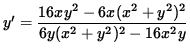.

Thus, the slope of the line tangent to the graph at the point (-1, 1) is,

and the equation of the tangent line is

y - ( 1 ) = (1) ( x - ( -1 ) )

or

y = x + 2 .

SOLUTION 11 : Begin with x2 + (y-x)3 = 9 . If x=1 , then

(1)2 + ( y-1 )3 = 9

so that

( y-1 )3 = 8 ,

y-1 = 2 ,

y = 3 ,

and the tangent line passes through the point (1, 3) . Now differentiate both sides of the original equation, getting

D ( x2 + (y-x)3 ) = D ( 9 ) ,

D ( x2 ) + D (y-x)3 = D ( 9 ) ,

2x + 3 (y-x)2 D (y-x) = 0 ,

2x + 3 (y-x)2 (y'-1) = 0 ,

so that (Now solve for y' .)

2x + 3 (y-x)2 y'- 3 (y-x)2 = 0 ,

3 (y-x)2 y' = 3 (y-x)2 - 2x ,

and.

Thus, the slope of the line tangent to the graph at (1, 3) is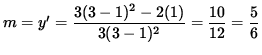,

and the equation of the tangent line is

y - ( 3 ) = (5/6) ( x - ( 1 ) ) ,

or

y = (5/6) x + (13/6) .

SOLUTION 12 : Begin with x2y + y4 = 4 + 2x . Now differentiate both sides of the original equation, getting

D ( x2 y + y4 ) = D ( 4 + 2x ) ,

D ( x2 y ) + D (y4 ) = D ( 4 ) + D ( 2x ) ,

( x2 y' + (2x) y ) + 4 y3 y' = 0 + 2 ,

so that (Now solve for y' .)

x2 y' + 4 y3 y' = 2 - 2x y ,

(Factor out y' .)

y' [ x2 + 4 y3 ] = 2 - 2x y ,

and

(Equation 1)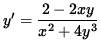.

Thus, the slope of the graph (the slope of the line tangent to the graph) at (-1, 1) is.

Since y'= 4/5 , the slope of the graph is 4/5 and the graph is increasing at the point (-1, 1) . Now determine the concavity of the graph at (-1, 1) . Differentiate Equation 1, getting.

Now let x=-1 , y=1 , and y'=4/5 so that the second derivative is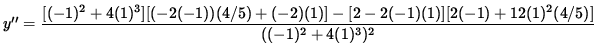.

Since y'' < 0 , the graph is concave down at the point (-1, 1) .